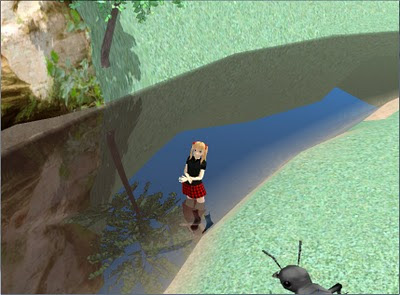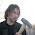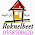## Tuesday, October 4, 2011

### Reflective water with GLSL, Part II

In the first part I explained how to implement a basic reflective surface. But as we could see, it was very poor approximation of true water, looking more like mirror lying on the ground.  First because real water is almost never completely still. But it is also not perfect mirror - depending on viewing angle we can see more or less into the water.
Before going to implementing ripples I'll show how to adjust water shaders so the angle-dependency of reflection strength is taken into account. And being already there, how to fake the light attenuation/scattering in water so it is not crystal-clear but has more realistic color.

1. The relation of reflectivity and viewing angle

If you imagine looking at water body from different angles it should be obvious, that the lower is viewing angle the more light it reflects. Sea may look almost like perfect mirror during tranquil sunset - but if you are looking daytime from the top of a cliff, you can see the blueish-greenish color of water and only a little reflection.

The reflectivity of water comes from the difference in refractive indexes of air and water. As the speed of light is different in these mediums, some light is reflected and the light entering water changes slightly its direction - the latter is called refraction. Refraction is another phenomenon that can add realism, but we will not try to simulate it here.A schematic diagram of reflection and refraction

Mathematically the amount of light reflected from the surface of water is described by Fresnel equations:Fresnel reflection equations (source Wikipedia)

Rs and Rt are the reflectance values of vertically and horizontally polarized light.
θi and θt are the angles between the surface normal and incident and refracted rays.
n1 and n2 are the refractive indices of two media - in our case air and water. The relevant values are:

n1 = 1.000277 ≈ 1
n2 = 1.3330

We do not need θt because it can be derived from θi and refractive indices using Snell's law - look at the rightmost part of equations.
The reflectance values are different for differently polarized light. This explains the magic behind anti-glare sunglasses and optical filters - they cut off vertically polarized light, that is much more strongly reflected.
It is also interesting to know that skylight is in fact somewhat polarized. But for our simulation we ignore this and treat all light as the uniform mix of both polarizations. In that case, the total reflectance can be simply calculated as:

R = (Rs + Rt) / 2

The full Fresnel equation above is a bit too complex for our shaders. It is physically correct - but our goal is natural or good-looking scene and we are ready to sacrify a little here ad there to save shader instructions for other things.
There is quite simple approximation available. Take a look at the following graph:Fresnel reflectance values Rs, Rp and R (blue, red, yellow) and our approximation (green)
The green line represents function:

R = Rmin + (1 - Rmin) * (1- cos θi)5

That can be used as good approximation.
Rmin is 0.02 for real water, but you may want to increase it to something like 0.1 or even more, unless you have very good HDR implementation. The problem is that real sky is really bright - if you are using dimmed down version of sky, its reflection is not visible at all from high angles.

That's it. Now we have reflectance value calculated - but we cannot yet update our shaders. Unlike in our previous lesson, where the reflection was all that had to be rendered, we now have to render the water itself too - unless the reflectance is exactly 1.

2. Rendering water

Water is normally dense medium and strongly scatters light. The actual formula is quite complex and depends on the amount of different chemical compounds (such as oxygen, humic acids) and various inorganic (such as clay) and organic (like plankton) particles in water. But our goal is not to simulate procedurally the color and turbidity of water, but instead find a meaningful set of descriptive parameters, that will give us good enough approximation.

Scattering changes the intensity of light (ray) in two ways:
• Out-scattering - if ray of light goes through medium, some fraction of light is scattered away from direct path and thus the final amount of light is lower.
• In-scattering - as light is scattered to all directions, some light from rays originally going other directions is scattered to the direction of the ray of interest.
Because of out-scattering the bottom of deep water body is dark. Because of in-scattering objects inside water seem "tinted" with water color.Scattering in water. A - in-scattering. B - out-scattering.
In addition to scattering there is one more phenomenon causing less light to come from water - it is internal reflection. As light hits the bottom of water body or objects inside it and goes upwards from there, some of it is mirrored back into water from the air-water phase surface.
We ignore this at moment and will use shortcut - instead of adding together the light coming from inside water and light from reflection, we draw the latter as semitransparent surface using reflectance as alpha value. Thus the higher is reflectance, the lower is the effect of light from inside water - which is generally correct, because the internal and external reflectance values are correlated.

How good our water formula can be depends on whether we can read the color and depth values of the bottom of water body or underwater objects in shader or not. Here I present a simple approach, that does not use them, but instead adds the color of water to the reflection image.

We ignore the exact scattering equations, that involve multiple integrals and instead combine the "tint" of water with the color of reflection (that is semitransparent now) and then render them as semitransparent surface over the already rendered bottom of water. For this I use a very simple formula:

Ctint = Cwater * (Omin + (1 - Omin) * sqrt (min (thickness / Dopaque, 1)))

Ctint is the color that water adds to objects (premultiplied)
Cwater is the color of opaque water column
Omin is the minimal opacity of water. It should be 0 for realistic simulation, but in reality values 0.1-0.2 give overall better effect.
Dopaque is the depth of water column, that becomes fully opaque. The reasonable value is 2 m for freshwater bodies - the smaller the better, as it helps to hide the lack of refraction.
thickness is the thickness of water in given direction until bottom or some underwater object is hit.

Calculating thickness is tricky. The technically correct way would be to trace ray in refracted direction until bottom (line AC in following figure) - but we cannot afford to do that.
If you can use depth buffer, you can ignore refraction and calculate the distance the original ray would cover underwater (line AD). This overestimates the thickness, but as the effect only becomes noticeable at low viewing angle, where reflection dominates, it should look quite good.
Here I will use even simpler approximation. Just find the depth of water under given point of surface (point B on following figure), and pretend that water has uniform depth (line AB1). It underestimates depth at the slopes directed away from viewer and overestimates at slopes directed to viewer, but if the bottom of water is reasonably smooth it is not too bad.A diagram of different possible methods for water thickness calculation
How to get the actual water depth under given point?
Recall the previous tutorial. We set the Z coordinate of vertex to 0 (i.e. flatten our object), but kept the full original vertex coordinate in interpolatedVertexDepth.
Thus if the object being rendered as water is actually the bottom of water body, it will render as flat surface, but we have access to the original Z coordinate of it. In other words - the water depth.
Another approach would be to encode water depth into another vertex attribute. It has some good points - like no need to separate the bottom of water body from other terrain and the possibility to hand-code depth.

Once we have calculated water thickness with whatever method applicable, we treat the tint color as another semitransparent layer, lying directly beneath the reflection layer. The final color and alpha values can be calculated by standard alpha blending formula:

C = Areflection * Creflection + (1 - Areflection) * Cwater
A = Areflection + (1 - Areflection) * Awater

Where C and A are color and alpha values.
If the resulting alpha is below 1, bottom or underwater objects are partially visible.

There is no need to change vertex shader.

```uniform mat4 o2v_projection_reflection;
uniform sampler2D reflection_sampler;
uniform vec3 eye_object;
uniform float min_opacity, opaque_depth, opaque_color;

varying vec3 interpolatedVertexObject;

void main()
{
// Vertex on water surface
vec3 surfaceVertex = vec3(interpolatedVertexObject.xy, 0.0);
// Reflection angle
vec3 vertex2Eye = normalize (eye_object - surfaceVertex );
float cosT1 = vertex2Eye.z;
// Reflectance
float c = 1.0 - cosT1;
float R = min_reflectivity + (1.0 - min_reflectivity) * c * c * c * c * c;

// Water density
float depth = -interpolatedVertexObject.z;
float thickness = depth / max (cosT1, 0.01);
float dWater = min_opacity + (1.0 - min_opacity) * sqrt (min (thickness / opaque_depth, 1.0));
// Premultiply
vec3 waterColor = opaque_color * dWater;

vec4 vClipReflection = o2v_projection_reflection * vec4(interpolatedVertexObject , 1.0);
vec2 vDeviceReflection = vClipReflection.st / vClipReflection.q;
vec2 vTextureReflection = vec2(0.5, 0.5) + 0.5 * vDeviceReflection;

vec4 reflectionTextureColor = texture2D (reflection_sampler, vTextureReflection);
// Framebuffer reflection can have alpha > 1
reflectionTextureColor.a = 1.0;

// Combine colors
vec3 color = (1.0 - R) * waterColor + R * reflectionTextureColor.rgb;
float alpha = R + (1.0 - R) * dWater;

gl_FragColor = vec4(color, alpha);}```

We have added another uniform (in addition to water color and opacity ones) - eye_object, that is simply camera position relative to water object local coordinate system.

And real-time image from Shinya:Simple scene from Shinya with partial reflection and water opacity

Now it is a bit better than last time - but still artificial and lifeless. Next time I show, how to make it live - i.e. add waves or ripples.

Part 1 - reflective surface

Have fun!

1.This comment has been removed by the author.

2.There are few errors in the code, but still this is a great tutorial, thanks!
Looking forward to Part III
*2.5 years have passed, but still .. ;-)

3.Yeah, sorry for not updating this for ages...
The truth is that I implemented some wave generators but was never fully satisfied with them. And then worked on completely other things and thus never wrote a follow-up.
But I hope to finish it one day still. And thank you for your interest.

1.Thx in advance, and plz help it would mean alot

2.What do shadows have to do with this Reflective Water tutorial?

4.Thanks for reply! I will see what I can do myself. I wish you good luck with your current interests! :)

5.ما تهتم به شركة كشف تسربات المياه بالرياض هو البحث عن كيفة علاج مشاكل تسريبات المياه التي تطرأ علي المكان فجأة بواسطة اجهزة الكشف الحدية التي تستخدمها شركة كشف تسربات بالرياض والتي تسعي للوصول الي افضل النتائج المثالية القادرة علي حل هذه المشكلة بدون تدمير فالاعتماد علي الاساليب الحديثة يساعدكم في الحصول علي نتيجة مثالية في مصلحة العميل فنحن لا نكتفي بتقديم هذه الاعمال في مدينة الرياض فقط بلا لدينا الفنين المتميزة الذي يقدمون شركة كشف تسربات المياه بالدمام التي تعمل علي حل مشكلة البيت بدون الاعتماد علي ا اساليب تقليدية التي تستخدما بعض مقدمي خدمة شركة كشف تسربات بالدمام فلا تتكايل بشأن هذا العمل بالذات لانه يحل لك الكثير من المشاكل

6.العاب بنات يحتوي موقعنا على تشكيلة من العاب تلبيس بنات متجددة باستمرار وكل مايتعلق بصنف العاب بنات تلبيس ومكياج والعاب طبخ ومرحبا بكم في العاب تلبيس
العاب بنات - العاب تلبيس
العاب طبخ al3ab-banat01

7.كيف تحقق النجاح بالنسبة لخدمات كشف تسربات المياه بجدة حتي نكون علي اقتناع تام بأننا شركة كشف تسربات بجدة تقدم خدمات لا مثيل لها من خلال فنين يمتلكون القدرة علي اكتشاف جميع انواع التسريبات من خلال تدريبهم العالي علي الاجهزة الحديثة التي تساعد علي ذلك بدون هدم او تكسير كل ذلك سوف تجدونه عندما تتواصلوا مع شركة كشف تسربات المياه بجدة والتي تقدم كل ما لديها لمساعدتكم

8.9.